Courses

# Test: Strain Energy And Resilience Level - 3

## 10 Questions MCQ Test Engineering Mechanics | Test: Strain Energy And Resilience Level - 3

Description
This mock test of Test: Strain Energy And Resilience Level - 3 for Civil Engineering (CE) helps you for every Civil Engineering (CE) entrance exam. This contains 10 Multiple Choice Questions for Civil Engineering (CE) Test: Strain Energy And Resilience Level - 3 (mcq) to study with solutions a complete question bank. The solved questions answers in this Test: Strain Energy And Resilience Level - 3 quiz give you a good mix of easy questions and tough questions. Civil Engineering (CE) students definitely take this Test: Strain Energy And Resilience Level - 3 exercise for a better result in the exam. You can find other Test: Strain Energy And Resilience Level - 3 extra questions, long questions & short questions for Civil Engineering (CE) on EduRev as well by searching above.
QUESTION: 1

### A simply supported beam of span ‘l’ and flexural rigidity EI carries a unit load at its mid-span section; the strain energy of the beam is

Solution: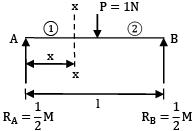U1 =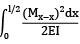=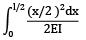=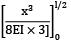U1 =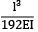U = 2U1 = 2 ×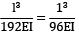QUESTION: 2

### A simply supported beam of span l carries a uniformly distributed load w/m over the entire span; the strain energy of the beam is given by

Solution: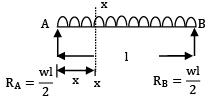Mx =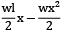U =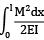=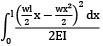=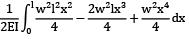=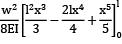=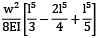=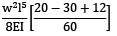=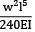QUESTION: 3

### The strain energy of stored due to bending for cantilever beam of span L, modulus of elasticity E, moment of inertia I and a point load P at the free end is

Solution: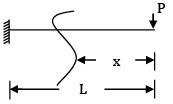U =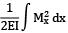=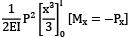=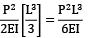QUESTION: 4

Total strain energy stored in a simply supported beam of span, 'L' and flexural rigidity 'EI 'subjected to a concentrated load 'W' at the centre is equal to

Solution: Strain energy

=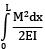=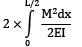=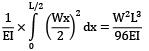Alternative method: In a funny way you may use Castigliano’s theorem,

δ =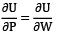We know that δ =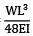for simply supported beam in concentrated load at mid span.

Then δ =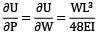or

U =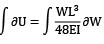partially integrating

with respect to W we ge U =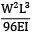QUESTION: 5

A steel specimen 150 mm2 in cross-section stretches by 0 · 05 mm over a 50 mm gauge length under an axial load of 30 kN. What is the strain energy stored in the specimen? (Take E = 200 GPa)

Solution: Strain Energy stored in the specimen

=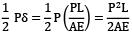=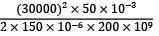= 0.75 N-m

QUESTION: 6

A cantilever beam, 2 m in length, is subjected to a uniformly distributed load of 5 kN/m. If E = 200 GPa and I = 1000 cm4, the strain energy stored in the beam will be

Solution:

U =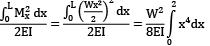=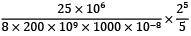= 10Nm

QUESTION: 7

A truck weighing 150 kN and travelling at 2 m/sec impacts with a buffer spring which compresses 1.25 cm per 10 kN. The maximum compression of the spring is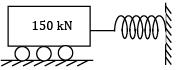Solution: Kinetic energy of the truck = strain energy of the string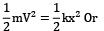x =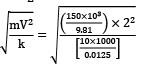= 0.2766m = 27.66cm

QUESTION: 8

A member formed by connecting a steel bar to an aluminium bar is shown in figure. Assuming that the bars are prevented from bucking sidewise, calculate the magnitude of the force P that will cause the total length of the member to decrease by 0.25 mm. The values of the elastic modulus for steel and aluminium are 2.1 × 105 N/mm2 and 0.7 × 105 N/mm2 respectively. What is the total work done by the force P?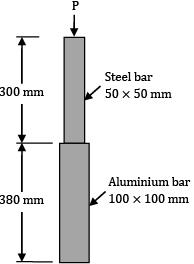(A) 27.5

(B) 28.5

Solution: Area of the steel bar = As = 50 × 50 = 2500 mm2

A!rea of aluminium bar = Aa = 100 × 100 = 10000 mm2

Total change in length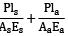= δ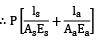= δ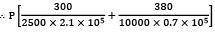= 0.25mm

P × 0.11143 × 10−5 = 0.25

∴ P = 224356 N

Total work done = 1/2 × load × deformation

=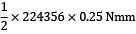= 28044 Nmm

= 28.044 Nm = 28.044 Joule

Question_Type: 5

QUESTION: 9

A 10 mm diameter mild steel bar of length 1.50 metres is stressed by a weight of 120 N dropping freely through 20 mm before commencing to stretch the bar. Find the maximum instantaneous stress and the elongation produced in the bar. (Take E = 2 × 105 N/mm2)

Solution: !rea of the bar = π / 4× (102) = 78.54 mm2

Let the maximum instantaneous stress be p N/mm2

∴ Maximum elongation = δl =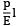Equating the loss of potential energy to the strain energy stored by the member, we have

P(h + δl) =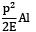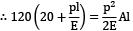∴ 2400 + 120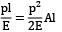∴ p2 − 240 p/A=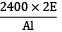∴ p2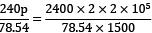∴ p2 − 3.056 p = 8148.714

∴ (p − 1.528)2 = 1848.714 + 2.334

∴ (p − 1.528)2 = 8151.049

∴ p − 1.528 = 90.283

p = 91.811 N/mm2

Alternatively, p =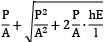But,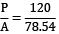= 1.528 N/mm2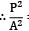= 1.5282 = 2.335

∴ p = 1.528 +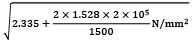= 91.811 N/mm2

∴ Maximum elongation

= δl =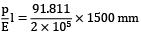= 0.6885 mm

QUESTION: 10

The L-shaped bar shown in the figure is of uniform cross-section 60 mm × 120 mm. Calculate the total strain energy. (Take E = 2 × 105 MPa, G = 0.8 × 105 MPa)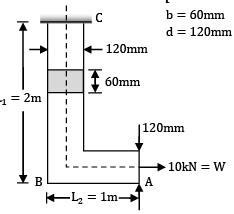Solution:

Total strain energy U = UB + US + UA = Strain energy due to bending of BC + Strain energy due to shear of BC + Strain energy due to axial load W

=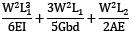Where, W = 10,000 N, L1 = 2000 mm,

L2 = 1000 mm, E = 2 × 105 MPa,

G = 0.8 × 105 MPa

I =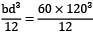=8.64 × 106 mm4

b = 60 mm, d = 120 mm

A = b × d = 60 × 120 = 7200 mm2

Putting the values Strain energy,

U =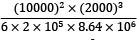+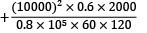+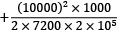= 77160.5 + 208.33 + 357.143 Nmm

= 77725.967 Nmm = 77.725 Nm